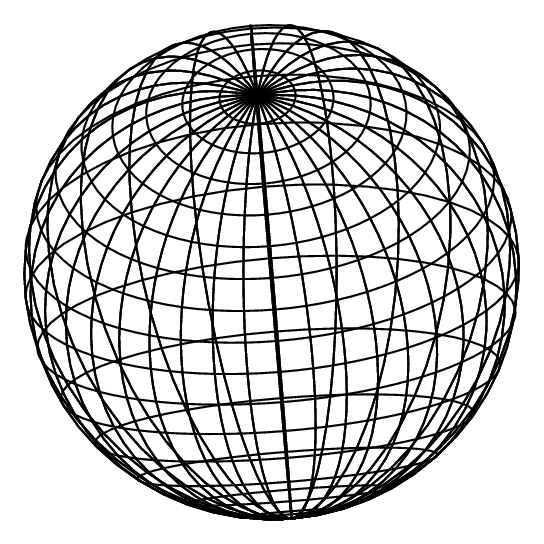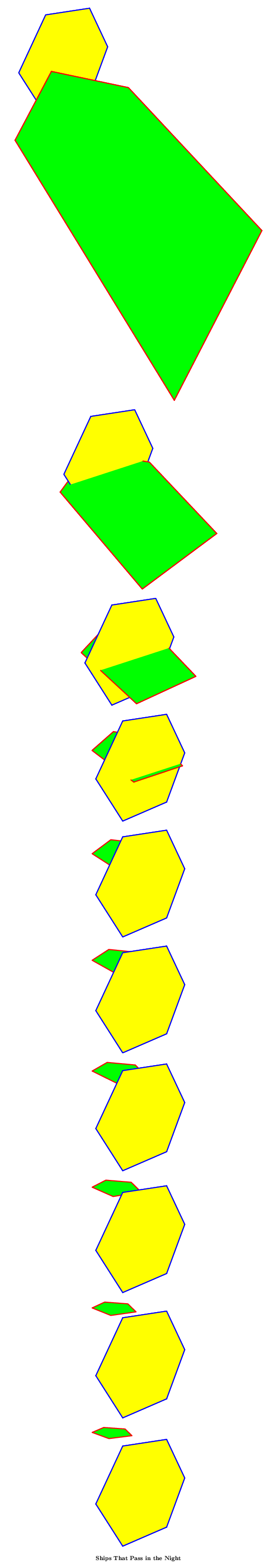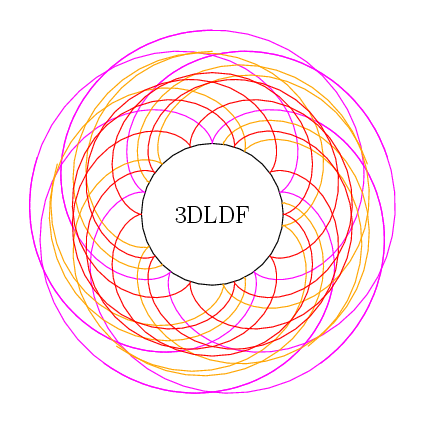# GNU 3DLDF Code Samples and Images

Author: Laurence D. Finston.

This copyright notice applies to the text and source code of this web site, and the graphics that appear on it. The software described in this text has its own copyright notice and license, which can be found in the distribution itself.

Copyright (C) 2003, 2004, 2005, 2006, 2007 The Free Software Foundation

Permission is granted to copy, distribute, and/or modify this document under the terms of the GNU Free Documentation License, Version 1.1 or any later version published by the Free Software Foundation; with no Invariant Sections, with no Front-Cover Texts, and with no Back-Cover Texts. A copy of this license is included in the file COPYING.TXT

Last updated: April 29, 2006

 Top Rotating Sphere Interpenetrating Polygons Sphere Epicycloid Pattern Contact

Back to main page

## Rotating Sphere

%% *** (3) Sphere. LDF 2005.05.02.

circle c[];
division_value := 64;
one_quarter_division_value := 1/4division_value;
three_quarters_division_value := 3/4division_value;
set c0 with_point_count division_value;
scale c0 (8, 0, 8);
rotate c0 by 90;
point p;
path q[];
p := get_point 0 c0;
q[division_value] += p;
pen thick_pen;
thick_pen := pencircle scaled (3.5, 3.5, 3.5);
pickup thick_pen;
step_value := 360 / division_value;
j := 1;
for i = 0 step step_value until 360 - step_value:
rotate c0 (0, step_value);
if (is_even j):
draw c0;
fi;
for k = 2 step 2 until one_quarter_division_value - 2:
q[k] += get_point (k) c0;
q[three_quarters_division_value + k]
+= get_point (three_quarters_division_value + k) c0;
endfor;
q[division_value] += get_point 0 c0;
j += 1;
endfor;
q[division_value] += cycle;
q[division_value] += ..;
draw q[division_value];
for i = 2 step 2 until one_quarter_division_value - 2:
q[i] += ..;
q[i] += cycle;
q[three_quarters_division_value + i] += ..;
q[three_quarters_division_value + i] += cycle;
draw q[i];
draw q[three_quarters_division_value + i];
endfor;
rotate current_picture by -25;
picture save_picture;
save_picture := current_picture;
clear current_picture;
message "Starting figures.";
for i = 1 upto 35:
rotate save_picture (0, 10);
beginfig(i);
output save_picture;
endfig;
message "Finished figure:";
show i;
endfor;
verbatim_metapost "bye;";
end;Back to contents
Back to main page

## Interpenetrating Polygons

The images in PDF format: ships_1.pdf

2005-04-07. The images in a compressed (gzipped) PostScript file: ships_1.ps.gz
I find this format to be the most convenient. By pressing the page down button repeatedly in Ghostview, or holding it down, one has the effect of flipping through animation drawings. I tried to generate an animated MNG file, but it failed when I tried to use more than 10 input files. I'll have to look into a better way of creating animations.

pickup pencircle scaled 3;
reg_polygon p;
reg_polygon q;
focus f;
set f with_position (-5, 10, -10) with_direction (-5, 10, 10) with_distance 10;
p := unit_pentagon scaled 5 rotated (0, 20) shifted (0, 0, -5);
q := unit_hexagon scaled 5 rotated (90, 20) shifted (0, 1, 3);
beginfig(0);
path r;
r := (-5, -22) -- (15, -22) -- (15, 7) -- (-5, 7) -- cycle;
undraw r;
output current_picture with_focus f;
verbatim_metapost "boxit.b(currentpicture); path p; p = bpath(b);";
clear current_picture;
endfig;
for i = 1 upto 10:
beginfig(i);
shift p (0, 0, 3);
filldraw p with_draw_color red with_fill_color green;
filldraw q with_draw_color blue with_fill_color yellow;;
verbatim_metapost "undraw p;";
endfig with_focus f with_surface_hiding;
endfor;
end;Back to contents
Back to main page

## Sphere

beginfig(1);
circle c[];

division_value := 64;
one_quarter_division_value := 1/4division_value;
three_quarters_division_value := 3/4division_value;

set c0 with_point_count division_value;
scale c0 (8, 0, 8);
rotate c0 by 90;

point p;
path q[];

q[division_value] += get_point 0 c0;

pen thick_pen;

thick_pen := pencircle scaled (2.5, 2.5, 2.5);

pickup thick_pen;

step_value := 360 / division_value;
j := 1;
for i = 0 step step_value until 360 - step_value:
rotate c0 (0, step_value);
if (is_even j):
draw c0;
fi;
for k = 2 step 2 until one_quarter_division_value - 2:
q[k] += get_point (k) c0;
q[three_quarters_division_value + k] += get_point (three_quarters_division_value + k) c0;
endfor;
q[division_value] += get_point 0 c0;
j += 1;
endfor;

q[division_value] += cycle;
q[division_value] += ..;
draw q[division_value];

for i = 2 step 2 until one_quarter_division_value - 2:
q[i] += ..;
q[i] += cycle;
q[three_quarters_division_value + i] += ..;
q[three_quarters_division_value + i] += cycle;
draw q[i];
draw q[three_quarters_division_value + i];
endfor;

rotate current_picture (-45, -15);

endfig;
end;Sphere

Back to contents
Back to main page

Back to contents